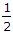# Civil Engineering - Applied Mechanics

1.
The centre of gravity of a homogenous body is the point at which the whole
volume of the body is assumed to be concentrated
area of the surface of the body is assumed to be concentrated
weight of the body is assumed to be concentrated
all the above.
Explanation:
No answer description is available. Let's discuss.

2.
Pick up the correct statement from the following :
If two equal and perfectly elastic smooth spheres impinge directly, they interchange their velocities.
If a sphere impinges directly on an equal sphere which is at rest, then a fraction(1 - e2) the original kinetic energy is lost by the impact.
If a smooth sphere impinges on another sphere, which is at rest, the latter will move along the line of centres.
If two equal spheres which are perfectly elastic impinge at right angles, their direction after impact will still be at right angles.
All the above.
Explanation:
No answer description is available. Let's discuss.

3.
Centre of gravity of a thin hollow cone lies on the axis of symmmetry at a height of
one-half of the total height above base
one-third of the total height above base
one-fourth of the total height above base
none of these.
Explanation:
No answer description is available. Let's discuss.

4.
A ball of mass 1 kg moving with a velocity of 2 m/sec collides a stationary ball of mass 2 kg and comes to rest after impact. The velocity of the second ball after impact will be
zero
0.5 m/sec
1.0 m/sec
2.0 m/sec.